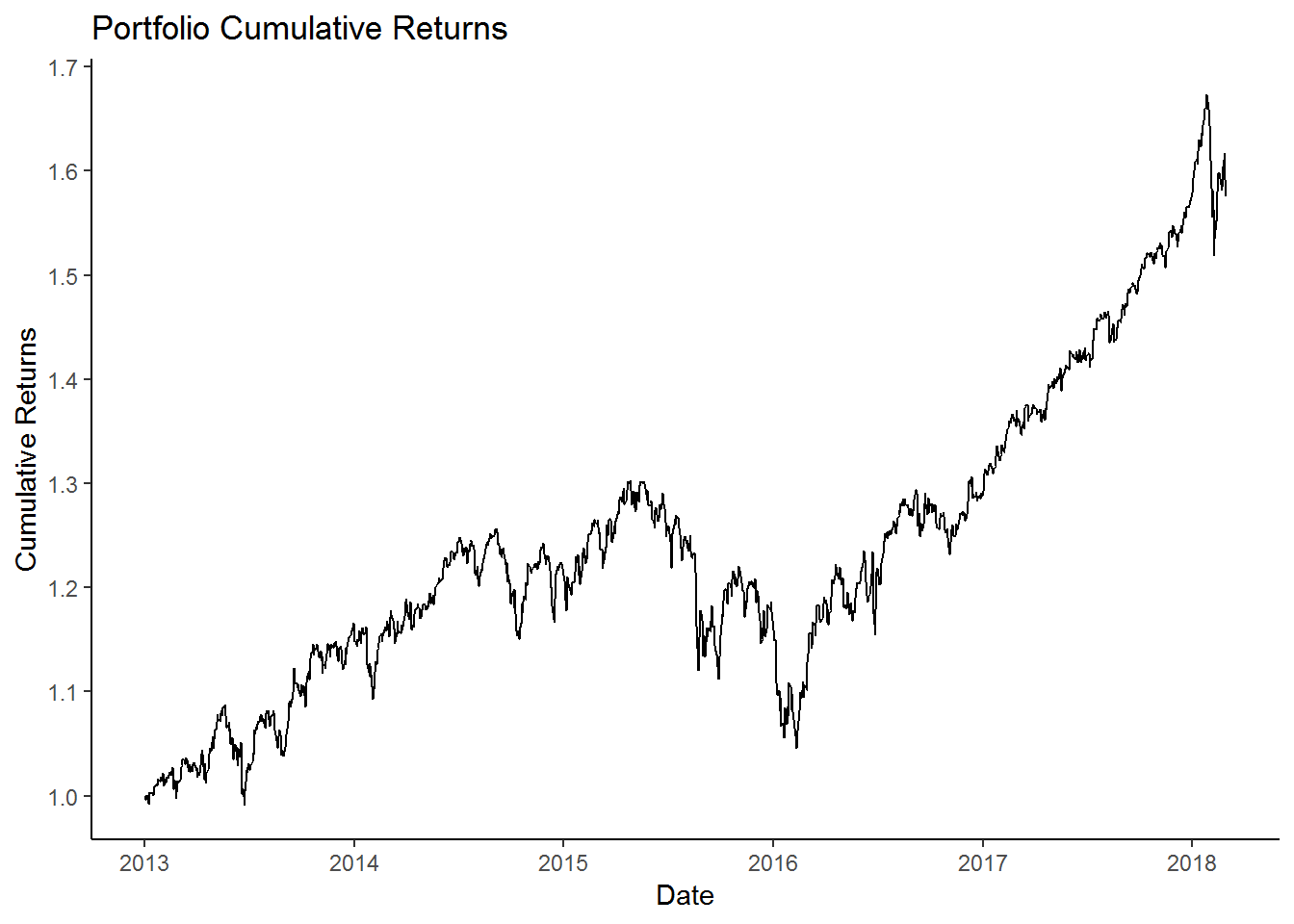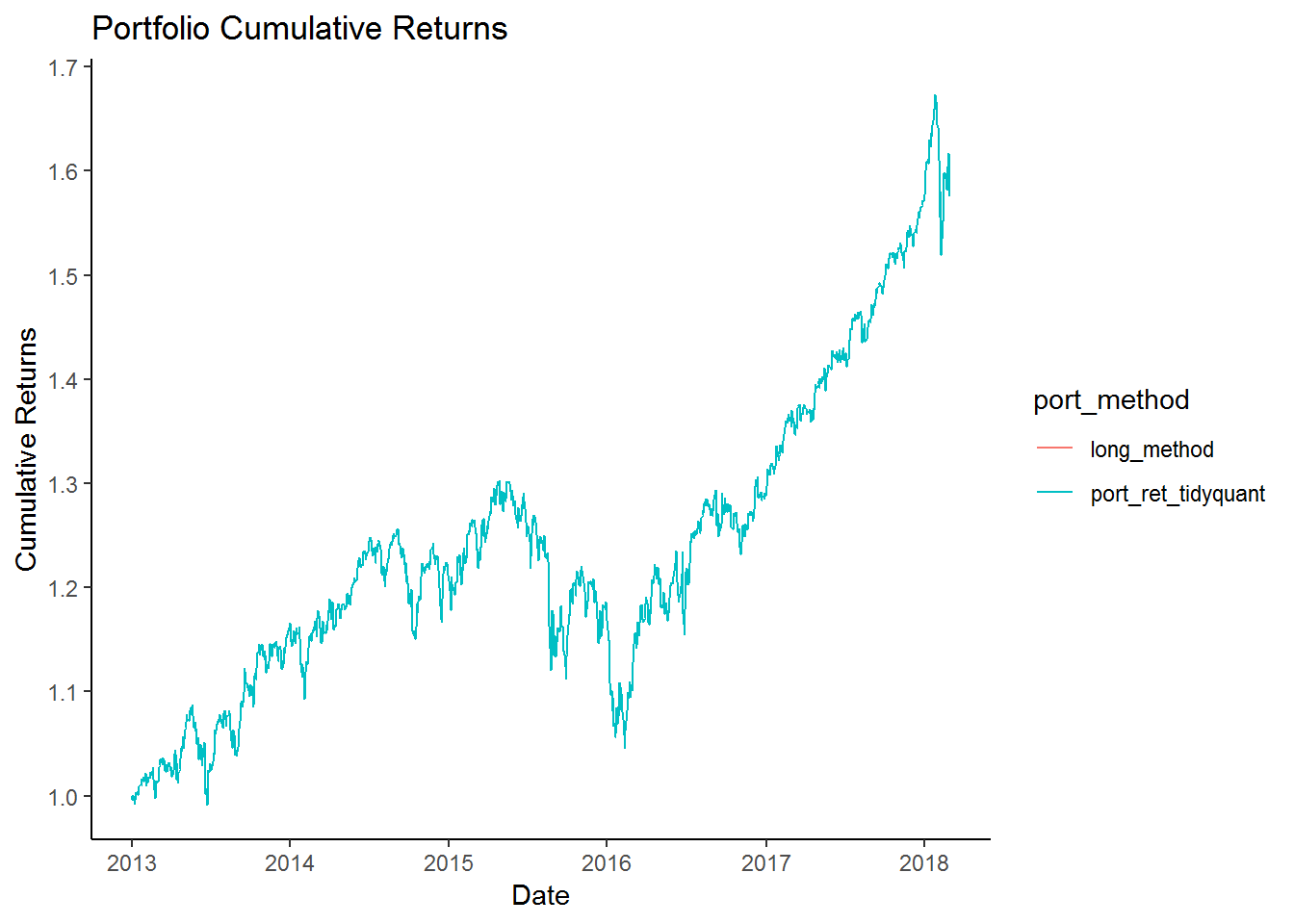## Calculating Cumulative portfolio returns in R

In the last post we learned how to construct a portfolio in R. We also learned how to calculate the daily portfolio returns. In this post we will learn how to calculate portfolio cumulative returns.

First lets load the library.

``library(tidyquant)``

Then lets load the ticker symbols for our assets that we will include in our portfolio.

``````# Asset tickers

tickers = c('BND', 'VB', 'VEA', 'VOO', 'VWO')``````

We will also create a vector for our asset weights.

``````# Asset weights

wts = c(0.1,0.2,0.25,0.25,0.2)``````

Next lets download the price data from yahoo finance.

``````price_data <- tq_get(tickers,
from = '2013-01-01',
to = '2018-03-01',
get = 'stock.prices')``````

Next we will calculate the daily returns for our assets.

``````ret_data <- price_data %>%
group_by(symbol) %>%
mutate_fun = periodReturn,
period = "daily",
col_rename = "ret")``````

For our ease of calculations, we will create a weight table.

``````wts_tbl <- tibble(symbol = tickers,
wts = wts)``````

Then we will join our weights table and the returns data.

``ret_data <- left_join(ret_data,wts_tbl, by = 'symbol')``

We will then calculate the weighted average of our asset returns.

``````ret_data <- ret_data %>%
mutate(wt_return = wts * ret)``````

Finally the portfolio returns are the sum of the weighted returns.

``````port_ret <- ret_data %>%
group_by(date) %>%
summarise(port_ret = sum(wt_return))``````

Once we have the portfolio returns, we will use the `cumprod()` function to calculate the cumulative returns for the portfolio.

``````port_cumulative_ret <- port_ret %>%
mutate(cr = cumprod(1 + port_ret))``````

We can visualize the portfolio returns using the below code.

``````port_cumulative_ret %>%
ggplot(aes(x = date, y = cr)) +
geom_line() +
labs(x = 'Date',
y = 'Cumulative Returns',
title = 'Portfolio Cumulative Returns') +
theme_classic() +
scale_y_continuous(breaks = seq(1,2,0.1)) +
scale_x_date(date_breaks = 'year',
date_labels = '%Y')``````We will post the entire code here.

``````library(tidyquant)

# Asset tickers

tickers = c('BND', 'VB', 'VEA', 'VOO', 'VWO')

# Asset weights

wts = c(0.1,0.2,0.25,0.25,0.2)

price_data <- tq_get(tickers,
from = '2013-01-01',
to = '2018-03-01',
get = 'stock.prices')

ret_data <- price_data %>%
group_by(symbol) %>%
mutate_fun = periodReturn,
period = "daily",
col_rename = "ret")

wts_tbl <- tibble(symbol = tickers,
wts = wts)
ret_data <- left_join(ret_data,wts_tbl, by = 'symbol')

ret_data <- ret_data %>%
mutate(wt_return = wts * ret)

port_ret <- ret_data %>%
group_by(date) %>%
summarise(port_ret = sum(wt_return))

port_cumulative_ret <- port_ret %>%
mutate(cr = cumprod(1 + port_ret))``````

But there is a simpler code if we use the tidyquant function. We will demonstrate how to use to tidyquant to calculate cumulative portfolio returns.

``````port_ret_tidyquant <- ret_data %>%
tq_portfolio(assets_col = symbol,
returns_col = ret,
weights = wts,
col_rename = 'port_ret',
geometric = FALSE)

port_cumulative_ret_tidyquant <- port_ret_tidyquant %>%
mutate(cr = cumprod(1 + port_ret))``````

As we can see that the above code is much shorter than the previous code. We can compare the two portfolio calculations.

``````port_cumulative_ret %>%
mutate(port_ret_tidyquant = port_cumulative_ret_tidyquant\$cr) %>%
select(-port_ret) %>%
rename(long_method = cr) %>%
gather(long_method,port_ret_tidyquant,
key = port_method,
value = cr) %>%
ggplot(aes(x = date, y = cr, color = port_method)) +
geom_line() +
labs(x = 'Date',
y = 'Cumulative Returns',
title = 'Portfolio Cumulative Returns') +
theme_classic() +
scale_y_continuous(breaks = seq(1,2,0.1)) +
scale_x_date(date_breaks = 'year',
date_labels = '%Y')``````We can see that both line overlap each other and the returns are the same. So in the future its best to use the shorter `tq_portfolio()` method.